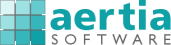http://www.aertia.com

Risk Simulator

by Real Options Valuation

Risk Simulator is a powerful Excel add-in software used for applying simulation, forecasting, statistical analysis, and optimization in your existing Excel spreadsheet models. It includes Monte Carlo simulation, optimization, statistical and analytical tools, and time-series and cross-sectional forecasting.

How do you make critical business decisions? Do you consider the risks of your projects and decisions, or are you more focused on returns? Do you have a hard time trying to understand what risk is, let alone quantifying risk? Well, our Risk Simulator software will help you identify, quantify, and value risk in your projects and decisions.

Risk Simulator is a powerful Excel add-in software used for applying simulation, forecasting, statistical analysis, and optimization in your existing Excel spreadsheet models. The software comprises four different modules:

• Monte Carlo Simulation
• Optimization
• Statistical and Analytical Tools
• Time-Series and Cross-Sectional Forecasting

Plus Risk Simulator is integrated with the Real Options Super Lattice Solver software, for solving strategic real options, financial options, and employee stock options.

Features

Monte Carlo Simulation

• 24 statistical distributions and one customizable empirical nonparametric distribution
• Complete integration with Excel (dynamic linking, VBA macros, and others)
• Comprehensive simulation and analytical reports for each functionality
• Correlated simulation with distributional truncation
• Multidimensional simulations with uncertain input parameters
• Simulation profiling for scenario analysis in simulation

Forecasting

• ARIMA models (time-series and panel)
• Auto-ARIMA models (time-series and panel)
• Basic Econometric Modeling (time-series and panel)
• Cubic Spline Forecasting (time-series and panel)
• Exponential J-Curves and Logistic S-Curves (time-series)
• GARCH Volatility Forecasts (time-series)
• Markov Chain Forecasts (time-series)
• Maximum Likelihood Models (cross-sectional)
• Multiple regression analysis (time-series, cross-sectional and panel)
• Nonlinear extrapolation (time-series)
• Stochastic process forecasting (time-series)
• Time-series analysis forecasting (time-series)

Optimization

• Optimization with continuous variables
• Optimization with discrete integer variables
• Optimization with mixed continuous and discrete variables
• Linear optimization
• Multiphasic optimization
• Nonlinear optimization
• Static optimization (fast single-point estimates)
• Dynamic optimization (simulation with optimization)
• Stochastic optimization (multiple iterations with distributions of decision variables)

Statistical and Analytical Tools

• Data Diagnostics (Autocorrelation, Correlation, Distributive Lags, Heteroskedasticity, Micronumerosity, Multicollinearity, Nonlinearity, Nonstationarity, Normality, Outliers, Stochastic Parameter Estimations)
• Data extraction and forecast extraction
• Distribution probability analysis (PDF, CDF, ICDF)
• Distributional fitting of existing data
• Hypothesis testing of distributions
• Nonparametric bootstrap simulation
• Scenario analysis
• Sensitivity analysis
• Statistical Analysis (Autocorrelation, Data Fitting, Descriptive Statistics, Hypothesis Tests, Nonlinear Extrapolation, Normality, Stochastic Parameter Estimation, Time-Series Forecasting)